0
5932

# RRB NTPC Syllogism Questions PDF

Download RRB NTPC Syllogism Questions and Answers PDF. Top 25 RRB NTPC Syllogism questions based on asked questions in previous exam papers very important for the Railway NTPC exam.Instructions

In the following question three statements are given, followed by two conclusions. You have to consider the statements to be true even if they seem to be at variance from commonly known facts. You have to decide which of the given conclusions, if any, follows from the given statements and select the appropriate option.

Question 1: Statement I: Some triangles are pentagons.
Statement II: All pentagons are circles.
Statement III: No circle is a hexagon. Conclusion I: No circle is a triangle.
Conclusion II: Some triangles can be hexagons.

a) Conclusion I only

b) Conclusion II only

c) Both the conclusions follow

d) Either conclusion I or conclusion II follows

e) None of the conclusions follows

Question 2: Statement I: Some chairs are stools.
Statement II: No stool is a table.
Statement III: All dusters are tables. Conclusion I: Some dusters can be chairs.
Conclusion II: All tables can be chairs.

a) Conclusion I only

b) Conclusion II only

c) Both the conclusions follow

d) Either conclusion I or conclusion II follows

e) None of the conclusions follows

Question 3: Statement I: All pens are markers.
Statement II: No pen is a pencil.
Statement III: Some pencils are markers. Conclusion I: All pencils can be markers.
Conclusion II: All markers can be pencils.

a) Conclusion I only

b) Conclusion II only

c) Both the conclusions follow

d) Either conclusion I or conclusion II follows

e) None of the conclusions followsInstructions

In these questions, the relationship between different elements is shown in the statements. These statements are followed by two conclusions. Study the conclusions based on the given statement(s) and select the appropriate answer.

Question 4: All cars are bikes.
Some boats are bikes.
No car is a boat. Conclusions: I. All bikes can be boats
II. All bikes cannot be cars

a) Only conclusion I follows

b) Only conclusion II follows

c) Either conclusion I or II follows

d) Neither of the conclusions follow

e) Both the conclusions follow

Instructions

In the following question three statements are given, followed by two conclusions. You have to consider the statements to be true even if they seem to be at variance from commonly known facts. You have to decide which of the given conclusions, if any, follows from the given statements and select the appropriate option.

Question 5: Statement I: Some doctors are engineers.
Statement II: All journalists are scientists.
Statement III: No engineer is a journalist. Conclusion I: All doctors can be journalists.
Conclusion II: Some engineers can be scientists.

a) Conclusion I only

b) Conclusion II only

c) Both the conclusions follow

d) Either conclusion I or conclusion II follows

e) None of the conclusions follows

Instructions

In the following question three statements are given, followed by two conclusions. You have to consider the statements to be true even if they seem to be at variance from commonly known facts. You have to decide which of the given conclusions, if any, follows from the given statements and select the appropriate option.

Question 6: Statement I: All students are boys.
Statement II: No girl is a teacher.
Statement III: Some teachers are students. Conclusion I: All students can be girls.
Conclusion II: Some boys are teachers.

a) Conclusion I only

b) Conclusion II only

c) Both the conclusions follow

d) Either conclusion I or conclusion II follows

e) None of the conclusions follows

Instructions

In the following question three statements are given, followed by two conclusions. You have to consider the statements to be true even if they seem to be at variance from commonly known facts. You have to decide which of the given conclusions, if any, follows from the given statements and select the appropriate option.

Question 7: Statement I: Some circles are quadrilaterals.
Statement II: All quadrilaterals are triangles.
Statement III: No triangle is a pentagon. Conclusion I: No triangle is a circle.
Conclusion II: Some circles can be pentagons.

a) Conclusion I only

b) Conclusion II only

c) Both the conclusions follow

d) Either conclusion I or conclusion II follows

e) None of the conclusions follows

Instructions

In the following question three statements are given, followed by two conclusions. You have to consider the statements to be true even if they seem to be at variance from commonly known facts. You have to decide which of the given conclusions, if any, follows from the given statements and select the appropriate option.

Question 8: Statement I: Some bats are balls.
Statement II: No wicket is a cap.
Statement III: Some caps are bats. Conclusion I: All bats can be wickets.
Conclusion II: All balls can be caps.

a) Conclusion I only

b) Conclusion II only

c) Both the conclusions follow

d) Either conclusion I or conclusion II follows

e) None of the conclusions follows

Instructions

In the following question three statements are given, followed by two conclusions. You have to consider the statements to be true even if they seem to be at variance from commonly known facts. You have to decide which of the given conclusions, if any, follows from the given statements and select the appropriate option.

Question 9: Statement I: All books are pens.
Statement II: Some pens are pencils.
Statement III: No pencil is a rubber. Conclusion I: Some rubbers can be books.
Conclusion II: No book is a pencil.

a) Conclusion I only

b) Conclusion II only

c) Both the conclusions follow

d) Either conclusion I or conclusion II follows

e) None of the conclusions followsInstructions

In the following question three statements are given, followed by two conclusions. You have to consider the statements to be true even if they seem to be at variance from commonly known facts. You have to decide which of the given conclusions, if any, follows from the given statements and select the appropriate option.

Question 10: Statement I: Some grapes are bananas.
Statement II: All bananas are pears.
Statement III: All pears are mangoes. Conclusion I: No mango is a grape.
Conclusion II: Some mangoes can be bananas.

a) Conclusion I only

b) Conclusion II only

c) Both the conclusions follow

d) Either conclusion I or conclusion II follows

e) None of the conclusions follows

Instructions

In the following question, two conclusions are given and three different statements are given in each of the options. Choose the option from which the given conclusions can be logically inferred.

Question 11: Conclusion I: All shirts are jeans.
Conclusion II: Some jeans are pants.

a) All shirts are pants. Some pants are jeans. No jeans is a shirt.

b) Some shirts are pants. All pants are jeans. Some jeans are shirts.

c) No shirt is a pant. Some pants are jeans. Some jeans are shirts.

d) Some shirts are pants. Some pants are jeans. Some jeans are shirts.

e) All shirts are pants. All pants are jeans. Some jeans are shirts.

Instructions

In the question three statements are given, followed by three conclusions. You have to consider the statements to be true even if they seem to be at variance from commonly known facts. You have to decide which of the given conclusions, if any, follows from the given statements and select the appropriate option.

Question 12: Statement I: All reds are blacks.
Statement II: All blacks are blues.
Statement III: Some reds are greens. Conclusion I: Some blacks are greens.
Conclusion II: Some greens are blues.
Conclusion III: All reds can be greens.

a) Conclusion I only

b) Conclusion I and II only

c) Conclusion III only

d) Conclusions I, II and III

e) None of the conclusions follow

Instructions

Directions for the following five questions: In each question below are given four statements followed by three conclusions numbered I, II, and III. You have to take the given statements to be true even if they seem to be at variance with commonly known facts and then, decide which of the given conclusions logically follow(s) from the given statements, disregarding commonly known facts.

Question 13: Statements
All bricks are cubes.
All stones are marbles.
All marbles are bricks.
All pebbles are stones. Conclusion:
I. Some pebbles are bricks.
II. Some stones are cubes.
III: Some stones are bricks.

a) Conclusion I follows.

b) Conclusion II follows.

c) Conclusion III follows.

d) Conclusion II, III follow.

e) Conclusion I, II, III follow.

Instructions

In the following question three statements are given, followed by two conclusions. You have to consider the statements to be true even if they seem to be at variance from commonly known facts. You have to decide which of the given conclusions, if any, follows from the given statements and select the appropriate option.

Question 14: Statement I: All rupees are dollars.
Statement II: No dollar is a rouble.
Statement III: Some roubles are euros. Conclusion I: Some rupees can be euros.
Conclusion II: All euros can be dollars.

a) Conclusion I only

b) Conclusion II only

c) Both the conclusions follow

d) Either conclusion I or conclusion II follows

e) None of the conclusions follows

Instructions

Directions for the following five questions: In each question below are given four statements followed by three conclusions numbered I, II, and III. You have to take the given statements to be true even if they seem to be at variance with commonly known facts and then, decide which of the given conclusions logically follow(s) from the given statements, disregarding commonly known facts.

Question 15: Statements:
Some fruits are veggies.
I. Some fruits can be Japanese.
II. Some veggies can be Japanese.
III: Some fruits are not Japanese.

a) Conclusion I, II, III follow.

b) Conclusion II, III follow.

c) Conclusion I, II follow.

d) Conclusion I, III follow.

e) Only conclusion II follows.

Instructions

In the following question three statements are given, followed by two conclusions. You have to consider the statements to be true even if they seem to be at variance from commonly known facts. You have to decide which of the given conclusions, if any, follows from the given statements and select the appropriate option.

Question 16: Statement I: All lakes are rivers.
Statement II: Some rivers are ponds.
Statement III: No pond is an ocean. Conclusion I: Some lakes can be oceans.
Conclusion II: All rivers cannot be oceans.

a) Conclusion I only

b) Conclusion II only

c) Both the conclusions follow

d) Either conclusion I or conclusion II follows

e) None of the conclusions follows

Instructions

In the question, three statements are given, followed by two conclusions, I and II. You have to consider the statements to be true even if it seems to be at variance from commonly known facts. You have to decide which of the given conclusions, if any, follows from the given statements.

Question 17: Statement 1: All markers are pens.
Statement 2: No pen is a protractor.
Statement 3: Some protractors are boxes.

Conclusion I: All pens may be boxes.
Conclusion II: Some markers are protractors.

a) Only conclusion I follows

b) Only conclusion II follows

c) Both the conclusions follow

d) Neither conclusion I nor II follows

e) Either conclusion I or II followsInstructions

In the question three statements are given, followed by three conclusions. You have to consider the statements to be true even if they seem to be at variance from commonly known facts. You have to decide which of the given conclusions, if any, follows from the given statements and select the appropriate option.

Question 18: Statement I: All rats are cats.
Statement II: All cats are dogs.
Statement III: No cat is a rabbit. Conclusion I: All rabbits can be dogs.
Conclusion II: Some dogs are cats.
Conclusion III: Some rats can be rabbits.

a) Conclusion I only

b) Conclusion I and II only

c) Conclusion III only

d) Conclusions II and III only

e) None of the conclusions follow

Instructions

In the question, three statements are given, followed by two conclusions, I and II. You have to consider the statements to be true even if it seems to be at variance from commonly known facts. You have to decide which of the given conclusions, if any, follows from the given statements.

Question 19: Statement 1: All circles are cubes.
Statement 2: Some cubes are cuboids.
Statement 3: All cuboids are cones. Conclusion I: Some cones are cubes.
Conclusion II: Some circles can be cones.

a) Only conclusion I follows

b) Only conclusion II follows

c) Both the conclusions follow

d) Neither conclusion I nor II follows

e) Either conclusion I or II follows

Instructions

In the following question, two conclusions are given and three different statements are given in each of the options. Choose the option from which the given conclusions can be logically inferred.

Question 20: Conclusion I: Some bees are flies.
Conclusion II: All wasps are ants.

a) No ant is a bee. All wasps are flies. All flies are wasps.

b) All ants are wasps. Some flies are bees. No bee is a wasp.

c) All wasps are bees. All bees are ants. No ant is a fly.

d) All bees are ants. Some wasps are flies. All wasps are bees.

e) Some wasps are bees. Some bees are ants. All flies are ants.From the diagram, we can see that some circle can be triangles. Hence, conclusion I doesn’t follow.
From the diagram, we can see that some triangles can be hexagons. Hence, conclusion II follows.
Therefore, option B is the correct answer.

From the diagram, we can see that some dusters can be chairs. Hence, conclusion I follows.
From the diagram, we can see that all tables can be chairs. Hence, conclusion II follows.
Therefore, option C is the correct answer.

From the diagram, we can see that all pencils can be markers hence conclusion I follows.
No pen is a pencil and all pens are markers. Hence, all markers can’t be pencils. Thus, conclusion II doesn’t follow.
Therefore, option A is the correct answer.

Since some bikes are cars and no car is a boat, all bikes cannot be boats. Thus, conclusion I is not possible.
Since some bikes are boats and no car is a boat, all bikes cannot be boats. Thus, conclusion II follows.
Hence, option B is the correct answer.

Since some doctors are engineers and no engineer is a journalist, all doctors cannot be journalists. Thus, conclusion I is not possible.

From the venn diagram, we can see that conclusion II is possible.
Hence, option B is the correct answer.

Since some students are teacher and no girl is a teacher, all students cannot be girls. Thus, conclusion I is not possible.

From the venn diagram, we can see that conclusion II follows.
Hence, option C is the correct answer.

From the diagram, we can see that some triangles can be circles hence, conclusion I doesn’t follow.
From the diagram, we can see that some circles can be pentagons hence, conclusion II follows.
Therefore, option B is the correct answer.

Some bats are caps and no cap is a wicket. So, all bats cannot be wickets. Thus, conclusion I is not possible.

From the venn diagram, we can see that conclusion II is possible.

Hence, option B is the correct answer.

From the diagram, we can see that some rubbers can be pencils hence conclusion I follows.
From the diagram, we can see that some books can be pencils hence conclusion II doesn’t follow.
Therefore, option A is the correct answer.

From the diagram, we can see that some grapes can be mangoes hence, conclusion I doesn’t follow.
From the diagram, we can see that some mangoes can be bananas hence, conclusion II follows.
Therefore, option B is the correct answer.

Venn diagram for option A:

From the venn diagram, we can see that only conclusion II follows.

Venn diagram for option B:

From the venn diagram, we can see that only conclusion II follows.

Venn diagram for option C:

From the venn diagram, we can see that only conclusion II follows.

Venn diagram for option D:

From the venn diagram, we can see that only conclusion II follows.

Venn diagram for option E:

From the venn diagram, we can see that both the conclusions follow.

Hence, option E is the correct answer.

From the venn diagram, we can see that all the conclusions follow.

Hence, option D is the correct answer.From the diagram, we can see that some pebbles are definitely bricks. Hence, conclusion I follows.
From the diagram, we can see that some stones are definitely cubes. Hence, conclusion II follows.
From the diagram, we can see that some stones are definitely bricks. Hence, conclusion III follows.
Hence we can say that option E is correct.

From the venn diagram, we can see that conclusion I follows.
Also, some roubles are euros and no dollar is a rouble. Thus, all euros cannot be dollars. Therefore, conclusion II does not follow.
Hence, option A is the correct answer.

From the diagram, we can see that some fruits can be Japanese. Hence, conclusion I follows.
From the diagram, we can see that some veggies can be Japanese. Hence, conclusion II follows.
All fruits can be Japanese. There is no restriction on that part. Hence conclusion III doesn’t follow.
Therefore, we can say that option C is correct.

From the venn diagram, we can see that conclusion I follows. Also, all rivers cannot be oceans because some rivers are ponds and no pond is an ocean. Thus, conclusion II also follows.
Hence, option C is the correct answer.

We can see that all pens can be boxes. Hence conclusion I follows.
We can see that no marker can be protractor. Hence conclusion II doesn’t follow.
Thus option A is the correct answer.

From the venn diagram, we can see that conclusions I and II are possible and conclusion III is not possible. Hence, option B is the correct answer.

We can see that some cones have to be cubes. Hence, conclusion I follows.
We can see that some circles can be cones. Hence, conclusion II follows.
Therefore, option C is the correct answer.

One of the possibilities of option A can be represented diagrammatically as follows: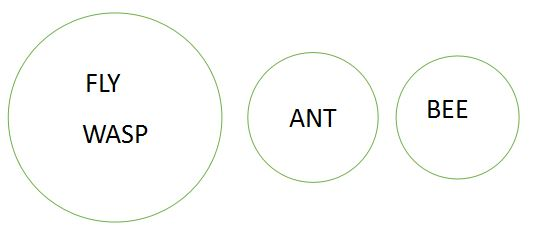As we can see, we cannot infer any of the conclusions. Therefore, we can eliminate option A.
Option B can be represented diagrammatically as follows without violating any of the conditions: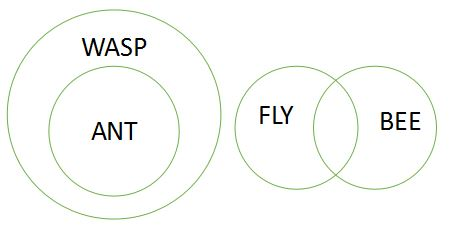As we can see, we can infer only conclusion I. Conclusion II cannot be inferred. Therefore, we can eliminate option B as well.

Option C can be represented diagrammatically as follows: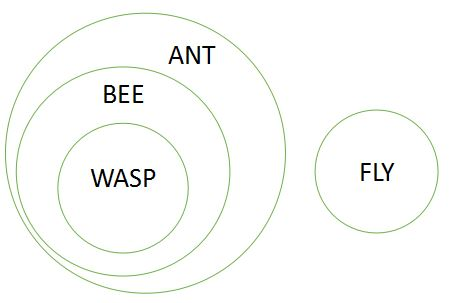Only conclusion II can be inferred from option C. Conclusion I cannot be inferred from the diagram given above. Therefore, we can eliminate option C too.

Option E can be represented diagrammatically as given below: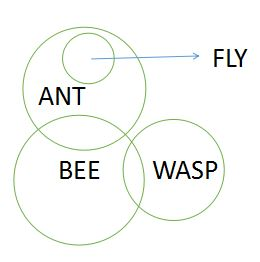Neither of the conclusions can be said to be definitely true. Therefore, we can eliminate option E.

Option D can be represented diagrammatically as follows:

As we can see, bees that are wasps are flies. Therefore, some bees are flies. Conclusion I is definitely true.
All wasps are ants. Therefore, conclusion II is also true. Both the conclusions are true for the statements given in option D. Therefore, option D is the right answer.Option A can be represented diagrammatically as follows: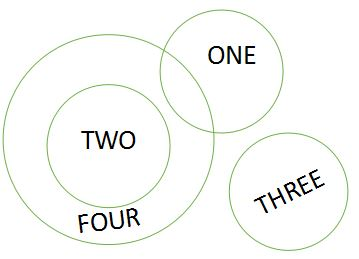Both the conclusions are possibilities. We cannot say either of the conclusions to be definitely true. Therefore, we can eliminate option A.

One of the possibilities of option B can be represented diagrammatically as follows: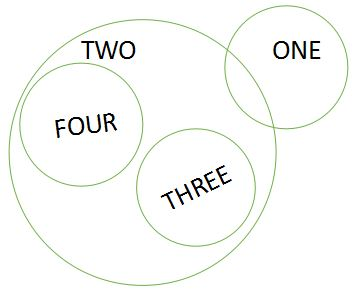As we can see, none of the conclusions can be inferred from option B.

Option C can be represented diagrammatically as follows:

Only conclusion II can be definitely said to be true. Conclusion I may or may not be true. Therefore, we can eliminate option D as well.

Option E can be represented diagrammatically as follows:

Option A can be represented diagrammatically as follows:

As we can see, we cannot ascertain that all highs are lows. Therefore, we can eliminate option C as well.

Option D can be represented diagrammatically as follows:

We cannot say with conviction that all highs are lows. Therefore, we can eliminate option E too.

Option B can be represented diagrammatically as follows:

On drawing all possible cases,

We can see that some erasers may or may not be books. Hence conclusion I doesn’t follow.

Since all pencils are erasers and some pens are pencils therefor some pens has to be erasers. Hence conclusion II follows.

Thus option B is the correct answer.Venn diagram for option A:

Venn diagram for option B:

Venn diagram for option C:

Venn diagram for option D:

Venn diagram for option E:

Hence, option B is the correct answer.